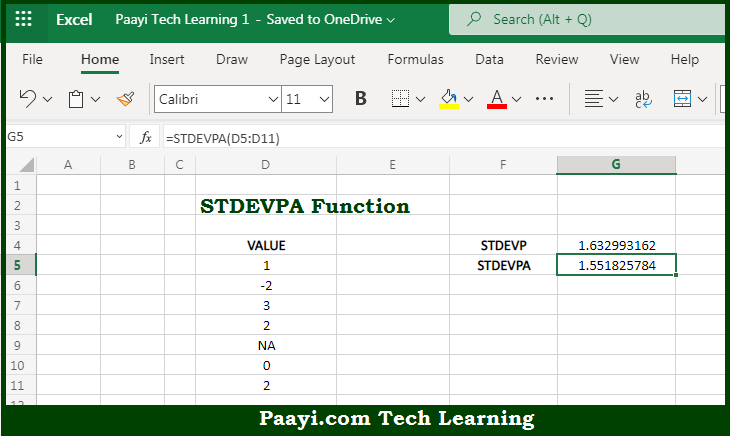# Learn How to Use Microsoft Excel STDEVPA Function

Written by | 0 Comments | 506 Views

In this article, you will learn how to use the Microsoft Excel STDEVPA function and its prime function in Microsoft Excel. You will also get to know the Microsoft Excel STDEVPA function return value and syntax with the help of some examples.

Microsoft Excel STDEVPA Function

The main purpose of the Microsoft Excel STDEVPA function is to get the standard deviation in the sample data set. That implies, with the help of the STDEVPA function you can able to return the standard deviation for the entire sample or population. It should be noted that the STDEVPA evaluates the text and the logical that appear in references, unlike the STDEVO function. In case you want to calculate the standard deviation for a sample, use STDEVA or STDEV.S. So, with the help of the STDEVPA function, you can able to get the standard deviation of the entire sample or population.

Return Value of STDEVPA Function

The return value will be the estimated standard deviation.

Syntax of STDEVPA Function

=STDEVPA(number1, [number2], ...)

Where the arguments:

• number1: This is the first number or reference in the sample.
• number2: This is the second number or reference in the sample (optional).

## How to Use Microsoft Excel STDEVPA Function?So we know that Microsoft Excel STDEVPA function you can able to get the standard deviation in the sample data set. That implies, with the help of the STDEVPA function you can able to return the standard deviation for the entire sample or population. It should be noted that the STDEVPA evaluates the text and the logical that appear in references, unlike the STDEVO function. In case you want you to calculate the standard deviation for a sample, use STDEVA or STDEV.S. So, with the help of the STDEVPA function, you can able to get the standard deviation of the entire sample or population.